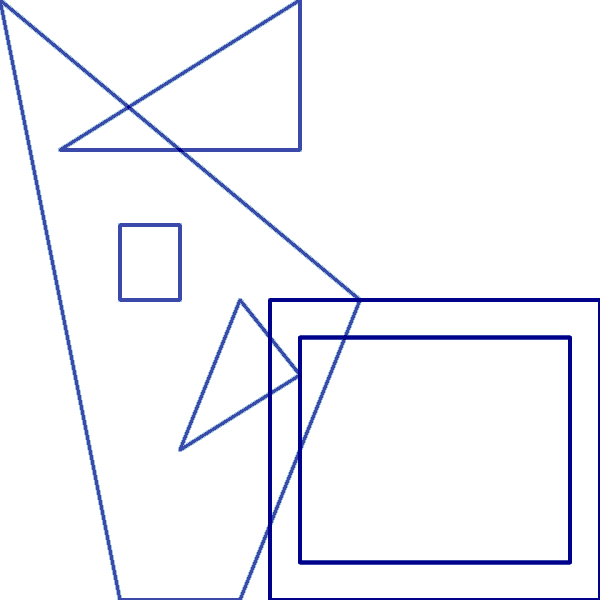# Polygons#

In addition to points, lines, areas, rasters, and trimeshes, Datashader can quickly render large collections of polygons (filled polylines). Datashader’s polygon support depends on data structures provided by the separate spatialpandas library, which extends Pandas and Parquet to support efficient storage and manipulation of “ragged” (variable length) data like polygons.

Before running these examples, you will need spatialpandas installed with pip:

```\$ pip install spatialpandas
```

or conda:

```\$ conda install -c pyviz spatialpandas
```
```import pandas as pd
import numpy as np
import colorcet as cc
import spatialpandas as sp
import spatialpandas.geometry
```

Pandas supports custom column types using an “ExtensionArray” interface. Spatialpandas provides two Pandas ExtensionArrays that support polygons:

• `spatialpandas.geometry.PolygonArray`: Each row in the column is a single `Polygon` instance. As with shapely and geopandas, each Polygon may contain zero or more holes.

• `spatialpandas.geometry.MultiPolygonArray`: Each row in the column is a `MultiPolygon` instance, each of which can store one or more polygons, with each polygon containing zero or more holes.

Datashader assumes that the vertices of the outer filled polygon will be listed as x1, y1, x2, y2, etc. in counter clockwise (CCW) order around the polygon edge, while the holes will be in clockwise (CW) order. All polygons (both filled and holes) must be “closed”, with the first vertex of each polygon repeated as the last vertex.

## Simple Example#

Here is a simple example of a two-element `MultiPolygonArray`. The first element specifies two filled polygons, the first with two holes. The second element contains one filled polygon with one hole.

```polygons = sp.geometry.MultiPolygonArray([
# First Element
[[[0, 0, 1, 0, 2, 2, -1, 4, 0, 0],         # Filled quadrilateral (CCW order)
[0.5, 1,  1, 2,  1.5, 1.5,  0.5, 1],     # Triangular hole (CW order)
[0, 2, 0, 2.5, 0.5, 2.5, 0.5, 2, 0, 2]], # Rectangular hole (CW order)

[[-0.5, 3, 1.5, 3, 1.5, 4, -0.5, 3]],],   # Filled triangle

# Second Element
[[[1.25, 0, 1.25, 2, 4, 2, 4, 0, 1.25, 0],          # Filled rectangle (CCW order)
[1.5, 0.25, 3.75, 0.25, 3.75, 1.75, 1.5, 1.75, 1.5, 0.25]],]]) # Rectangular hole (CW order)
```

The filled quadrilateral starts at (x,y) (0,0) and goes to (1,0), then (2,2), then (-1,4), and back to (0,0); others similarly go left to right (but drawing in either CCW or CW order around the edge of the polygon depending on whether they are filled or holes).

Since a `MultiPolygonArray` is a pandas/dask extension array, it can be added as a column to a `DataFrame`. For convenience, you can define your DataFrame as `sp.GeoDataFrame` instead of `pd.DataFrame`, which will automatically include support for polygon columns:

```df = sp.GeoDataFrame({'polygons': polygons, 'v': range(1, len(polygons)+1)})
df
```
polygons v
0 MultiPolygon([[[0.0, 0.0, 1.0, 0.0, 2.0, 2.0, ... 1
1 MultiPolygon([[[1.25, 0.0, 1.25, 2.0, 4.0, 2.0... 2

Polygons are rasterized to a `Canvas` by Datashader using the `Canvas.polygons` method. This method works like the existing glyph methods (`.points`, `.line`, etc), except it does not have `x` and `y` arguments. Instead, it has a single `geometry` argument that should be passed the name of a `PolygonArray` or `MultiPolygonArray` column in the supplied `DataFrame`. For comparison we’ll also show the polygon outlines rendered with `Canvas.line` (which also supports geometry columns):

```%%time
cvs = ds.Canvas()
agg = cvs.polygons(df, geometry='polygons', agg=ds.sum('v'))
float(agg.min()), float(agg.max())
```
```CPU times: user 4.59 s, sys: 34.8 ms, total: 4.62 s
Wall time: 4.62 s
```
```(1.0, 3.0)
```
```%%time
cvs = ds.Canvas()
agg = cvs.line(df, geometry='polygons', agg=ds.sum('v'), line_width=4)
float(agg.min()), float(agg.max())
```
```CPU times: user 3.77 s, sys: 19.7 ms, total: 3.79 s
Wall time: 3.79 s
```
```(0.0032808413085092525, 3.0)
```
```tf.Images(filled, unfilled)
```Here as you can see each polygon is filled or outlined with the indicated value from the `v` column for that polygon specification. The `sum` aggregator for the filled polygons specifies that the rendered colors should indicate the sum of the `v` values of polygons that overlap that pixel, and so the first element (`v=1`) has an overlapping area with value 2, and the second element has a value of 2 except in overlap areas where it gets a value of 3. Each plot is normalized separately, so the filled plot uses the colormap for the range 1 (light blue) to 3 (dark blue), while the outlined plot ranges from 1 to 2.

You can use polygons from within interactive plotting programs with axes to see the underlying values by hovering, which helps for comparing Datashader’s aggregation-based approach (right) to standard polygon rendering (left):

```import holoviews as hv